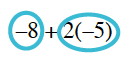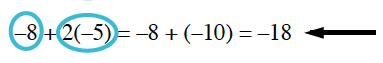### Home > CC2MN > Chapter 3 > Lesson 3.1.2 > Problem3-20

3-20.

Copy each expression below and circle each term. Then simplify each expression.

1. $-8+2(-5)$Begin by circling each term. Circles around the negative 8 and around 2 times negative 5.

Simplify the expression. Added to the expression is the following, = negative 8 + negative 10 = negative 18

$-18$

1. $3(7.5+2)+4.6$

Follow the steps outlined in part (a).

$33.1$ Remember to circle each term!

1. $4\frac{1}{2}(-2+1+7)$

Follow the steps outlined in part (a).

1. $5(6+2)+4+2(-5+8)$

Follow the steps outlined in part (a).